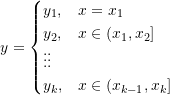### Piecewise Interpolation: Zero Degree Spline Interpolation

### 0 Degree Spline Interpolation

Given a set of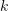data points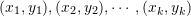, a spline of degree 0 can be defined as: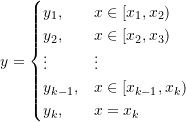In the previous spline definition, the interpolated value in the interval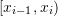is equal to the data value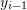. Alternatively, the interpolated value in the interval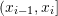can be set equal to the data value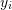. In this case,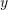can be defined as follows: